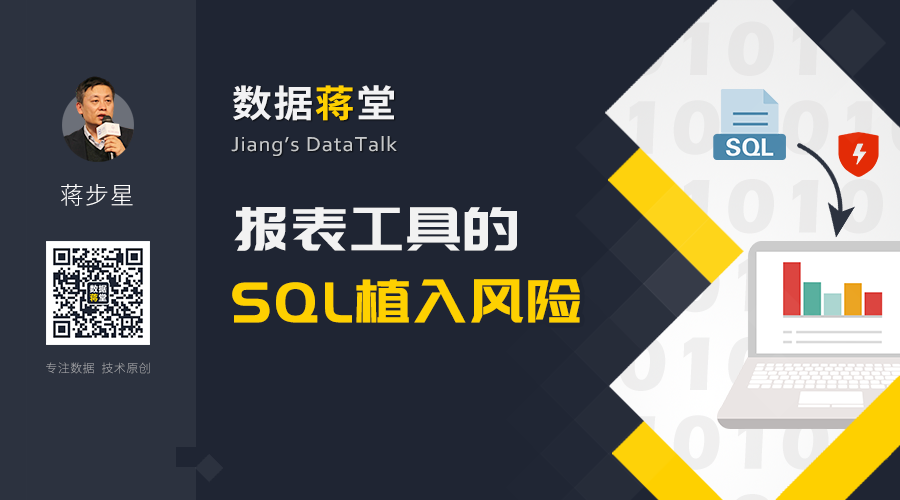# 报表工具的SQL植入风险SELECT ... FROM T WHERE \${w}

1=0 UNION SELECT ... FROM user

SELECT ... FROM T WHERE 1=0 UNION SELECT ... FROM user

SELECT ... FROM T WHERE (\${w})

SELECT ... FROM T WHERE (1=0 UNION SELECT ... FROM user)

1=0) UNION SELECT ... FROM user WHERE (1=1

SELECT ... FROM T WHERE (1=0) UNION SELECT ... FROM user WHERE (1=1)

SELECT ... FROM T WHERE (\${w}) OR \${w}

SELECT ... FROM T WHERE (1=0) UNION SELECT ... FROM user WHERE (1=1) OR 1=0) UNION SELECT ... FROM user WHERE (1=1